Hello guys,

Today I’m going to show you how to use Matlab code in any .Net platform such as Web.API, ASP.Net MVC, Console or WPF application.

Matlab, developed by MathWorks, has been using as a fourth generation programming language in computer science, especially academic world. You can write a complex calculation algorithm in a single code in Matlab, and that’s why Matlab is so popular in these sector. Furthermore, Matlab is more powerful, stable, reliable and faster then OpenCV or this kind of open source computer vision, neural network library. The major reason of this, Matlab is a commercial programming language for sure! 🙂 However, you may use Matlab under academic licence if your university has.

Anyway, let’s get started to build our WEB.API using Matlab code…

I had to change to use the latest version of Matlab, R2015b, when I was master’s degree student. You probably understand why I need to convert Matlab codes to .Net :p Because of my Master’s Thesis and its project! 😀

To be honest with you, it wasn’t so simple. However, if you read my article carefully, you can do this without any trouble.

Shall we begin?

OK, let’s create our first Matlab function like that:

```
function centers = FindCenters(I)
sens = 0.92; %Sensitivity
et = 0.03; %EdgeThreshold
end
```

I have a function, named FindCenters which finds center points of circles in an image that you pass function as an “I” parameter.

Click “Apps” tab in your Matlab 2015b. You now able to see “Application Compiler”, click and open it.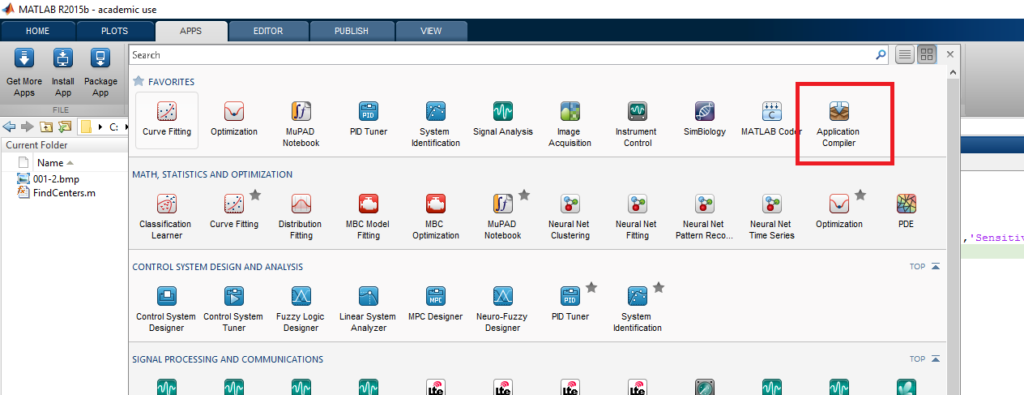When you open it first, you see this screen.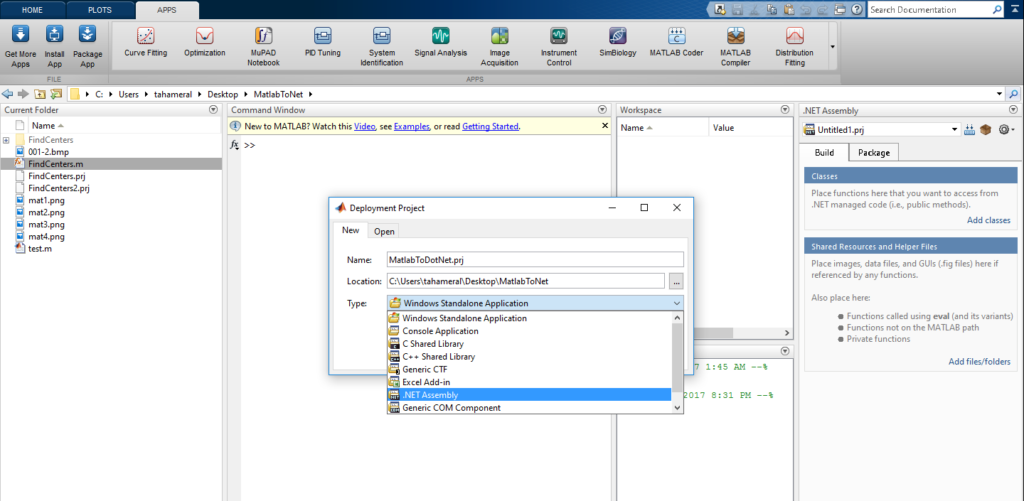Name your project name, choose your project’s location, then choose deployment project type, which is .Net Assembly in our case. If you don’t see .Net assembly option in your dropdown list, your matlab version or licence is not suitable for this.  Please make sure that you use the original Matlab!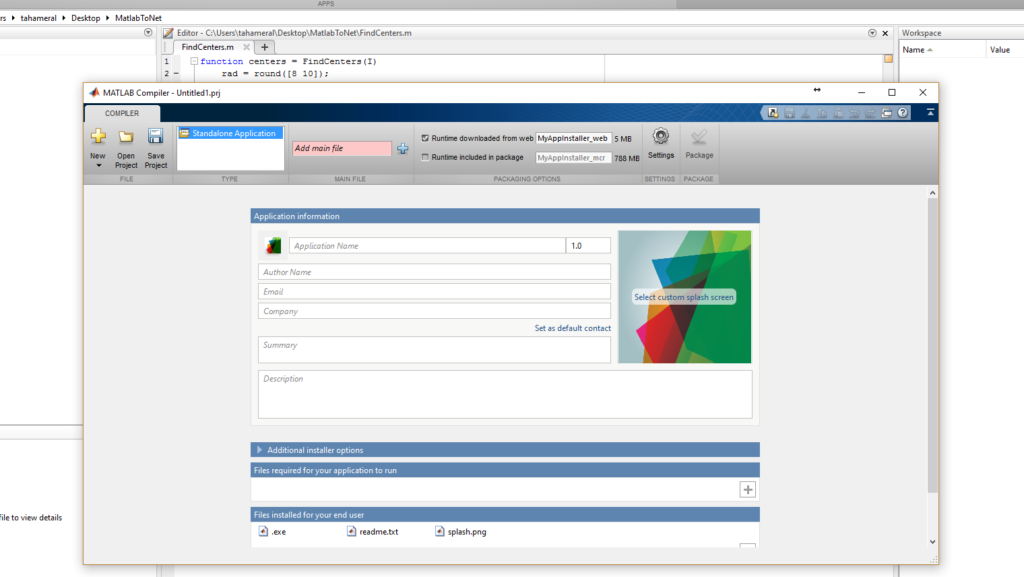Please note that, as of R2015a, the MATLAB Builder EX functionality for desktop applications has been merged with MATLAB Compiler. MATLAB Compiler requires MATLAB. MATLAB Builder JA, MATLAB Builder NE, the MATLAB Builder EX functionality for MATLAB Production Server, and the shared library `(.dll)` functionality of MATLAB Compiler have been merged into a new product named MATLAB Compiler SDK. MATLAB Compiler SDK requires MATLAB Compiler.

Click OK. Then you see .Net Assembly section on the right side of Matlab. Name your Class, and add files into this class. For our case, our file is FindCenters.m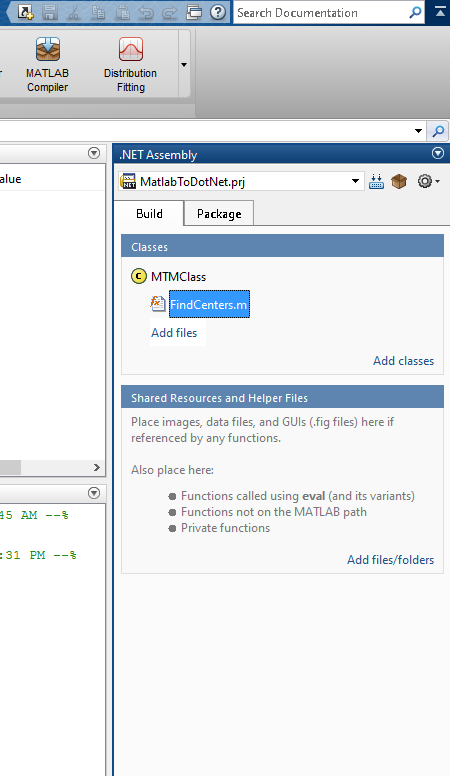When you have a look package tab, you would see the .dlls and other files that will generate at the end of building.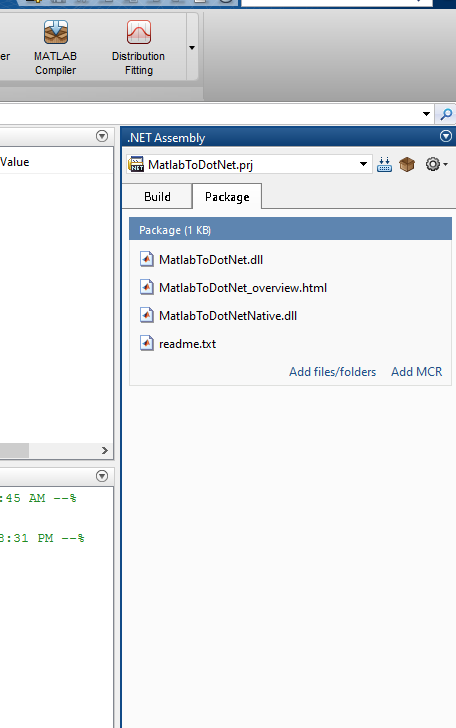If you set everything, click the build button that is located on the just right of the project name (MatlabToDotNet.prj)

As you can see on the image below, building started.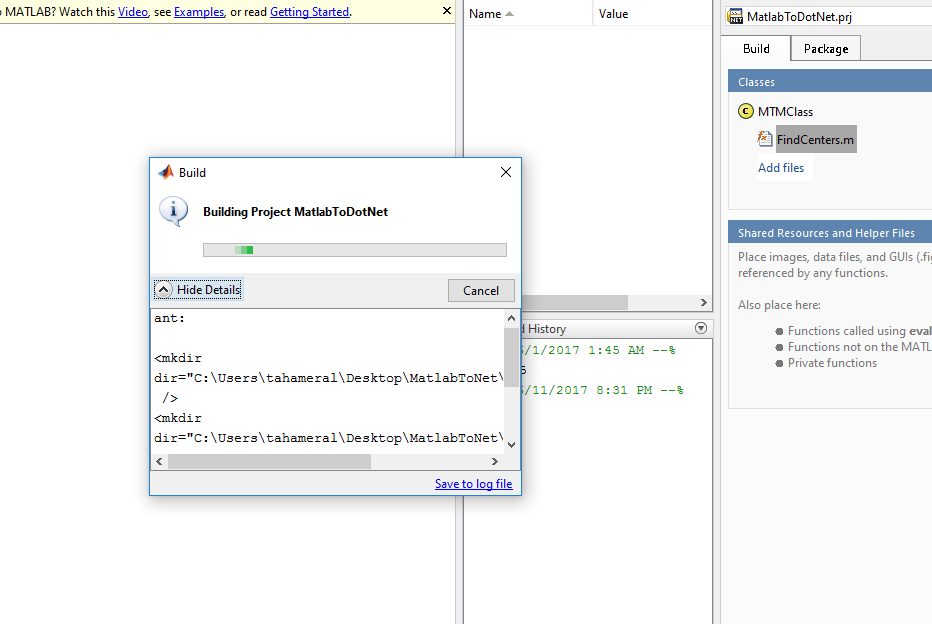After a couple of minutes later, building finished. When you have a look the building project folder, you would see 2 folders, which are src and distrib. We will use “MatlabToDotNet.dll” in the distrib folder.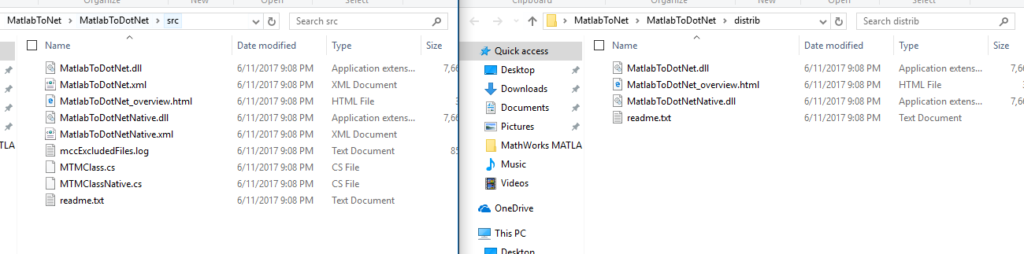But hold on! We haven’t finished yet! 🙂 We need to MWArray.dll for using Matlab style arrays, which are basically matrix. Find your own MWArray.dll in your Matlab installation directory. It is basically located in under C:\Program Files\MATLAB\R2015b\toolbox\dotnetbuilder\bin\win32\v2.0 folder.

Please make sure that you use 32 bit version of Matlab. Otherwise, you might get lots of errors when .Net WEB.API builds.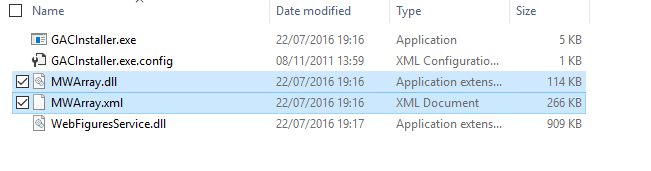Now we are ready to use our lovely dlls 🙂

Open your Visual Studio, and let’s create a new WEB.API project. File > New > Project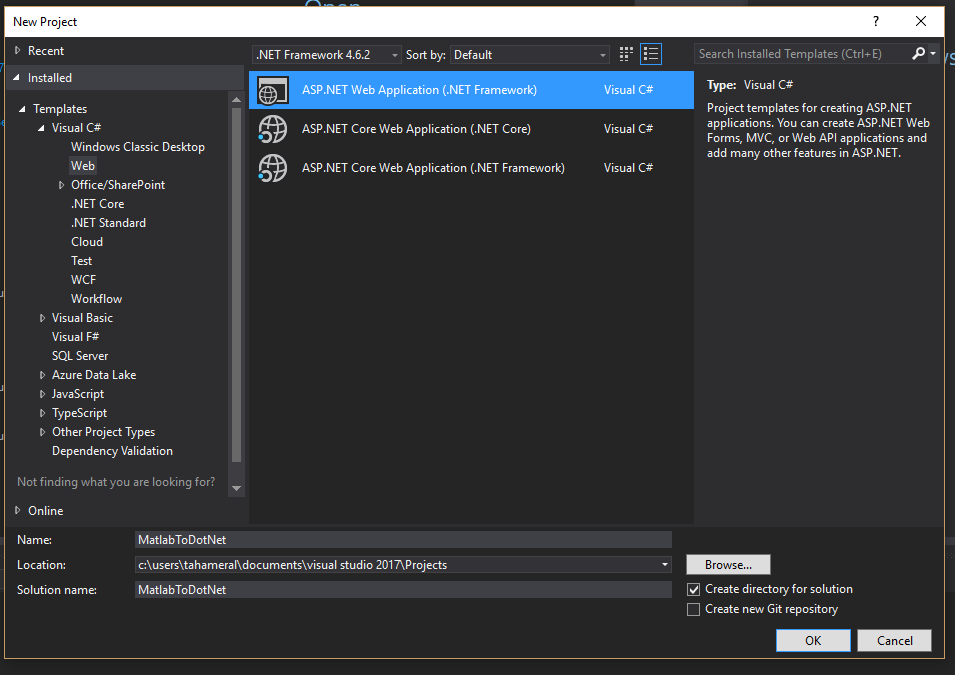Select WEB API or MVC.

Right click on the references, click browse and choose your dll that you generated, and MWArray.dll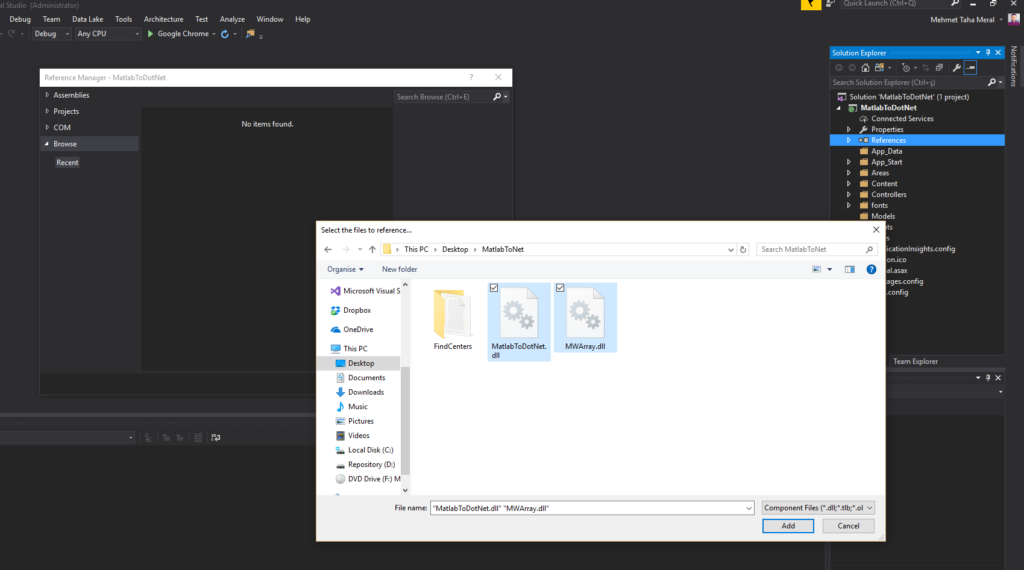Now let’s change the target platform. Right click on the project name, and click the properties. Find the platform target dropdown in the “build” section. Change it x86 instead of AnyCPU.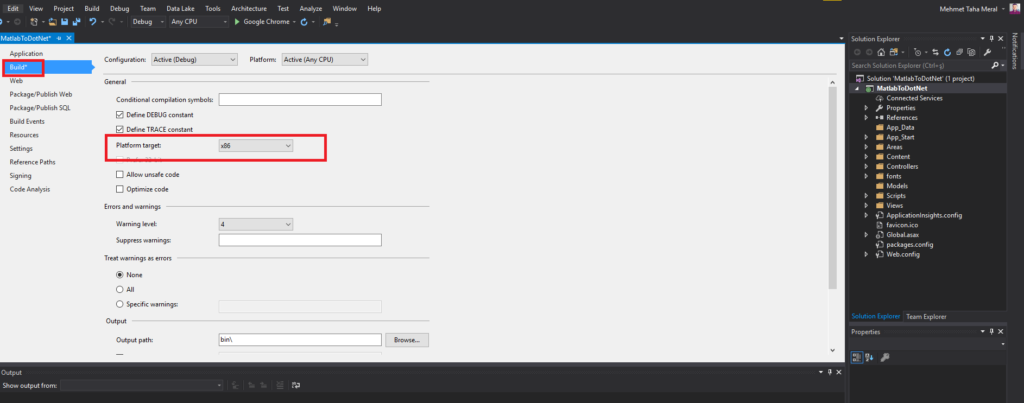Create your own new Web.API controller or just start from HomeController straight away.

```
using MatlabToDotNet;
using MathWorks.MATLAB.NET.Arrays;

[ResponseType(typeof(YourResultModel))]
public IHttpActionResult PostDetect(YourDetectModel dm)
{
// handling and converting image here

// handling and converting image here

//Get image dimensions
int width = bitmap.Width;
int height = bitmap.Height;

//Declare the double array of grayscale values to be read from "bitmap"
double[,] bnew = new double[height, width];

//Loop to read the data from the Bitmap image into the double array
int i, j;
for (i = 0; i &amp;lt; width; i++)
{
for (j = 0; j &amp;lt; height; j++)
{
Color pixelColor = bitmap.GetPixel(i, j);
double b = pixelColor.GetBrightness(); //the Brightness component

//Note that rows in C# correspond to columns in MWarray
bnew.SetValue(b, j, i);
}
}
MatlabToDotNet.MTMClass detectDots = null;
MWArray[] res = new MWArray;
MWNumericArray arr = bnew;
double[,] CirclePoints = null;

try
{
detectDots = new MatlabToDotNet.MTMClass();

// res contains whatever you return back on matlab function. If you return 3 different object, you can access the result through res, res, res. If so you have to call like&nbsp;.FindCenters(3, arr); 3 means the number of args out.
res = detectDots.FindCenters(1, arr);

// in our case, we just returned circlepoints, so:
CirclePoints = (double[,])((MWNumericArray)res).ToArray(MWArrayComponent.Real),

}
catch (Exception ex)
{
return NotFound();
}